﻿ 时滞高阶多智能体系统的一致性 Higher-Order Consensus in Multi-Agent System with Delay

Vol.06 No.09(2017), Article ID:23073,7 pages
10.12677/AAM.2017.69133

Higher-Order Consensus in Multi-Agent System with Delay

Hao Wen, Xiao Wang

College of Liberal Arts and Sciences, National University of Defense Technology, Changsha Hunan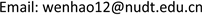Received: Nov. 24th, 2017; accepted: Dec. 12th, 2017; published: Dec. 19th, 2017ABSTRACT

In this paper, the consensus problem of high-order multi-agent system with delay is considered. Via constructing Lyapunov function, the result of high-order multi-agent system consensus is obtained when the delay is smaller than a certain value. Finally, a numerical simulation is used to verify our main result.

Keywords:Multi-Agent System, Delay, Consensus, Lyapunov Function1. 引言

$\left\{\begin{array}{l}{\stackrel{˙}{\xi }}_{i}^{1}\left(t\right)={\xi }_{i}^{2}\left(t\right)\hfill \\ {\stackrel{˙}{\xi }}_{i}^{2}\left(t\right)={\xi }_{i}^{3}\left(t\right)\hfill \\ \text{\hspace{0.17em}}\text{\hspace{0.17em}}\text{\hspace{0.17em}}\text{\hspace{0.17em}}\text{\hspace{0.17em}}\text{\hspace{0.17em}}\text{\hspace{0.17em}}\text{\hspace{0.17em}}\text{ }\text{\hspace{0.17em}}⋮\hfill \\ {\stackrel{˙}{\xi }}_{i}^{m}\left(t\right)={u}_{i}\left(t\right),\text{ }i=1,2,\cdots ,N.\hfill \end{array}$ (1)

${u}_{i}\left(t\right)=c\sum _{j=1,j\ne i}^{N}{G}_{ij}\sum _{k=1}^{m}{\alpha }_{k}\left({\xi }_{j}^{k}\left(t\right)-{\xi }_{i}^{k}\left(t\right)\right),\text{ }i=1,2,\cdots ,N.$ (2)

$\left\{\begin{array}{l}{\stackrel{˙}{\xi }}_{i}^{1}\left(t\right)={\xi }_{i}^{2}\left(t\right)\hfill \\ {\stackrel{˙}{\xi }}_{i}^{2}\left(t\right)={\xi }_{i}^{3}\left(t\right)\hfill \\ \text{\hspace{0.17em}}\text{\hspace{0.17em}}\text{\hspace{0.17em}}\text{\hspace{0.17em}}\text{\hspace{0.17em}}\text{\hspace{0.17em}}\text{\hspace{0.17em}}\text{\hspace{0.17em}}\text{\hspace{0.17em}}\text{ }⋮\hfill \\ {\stackrel{˙}{\xi }}_{i}^{m}\left(t\right)={u}_{i}\left(t-\tau \right),\text{ }i=1,2,\cdots ,N.\hfill \end{array}$ (3)

2. 预备知识及引理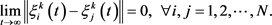${u}_{i}\left(t-\tau \right)=-c\sum _{j=1}^{N}{L}_{ij}\sum _{k=1}^{m}{\alpha }_{k}{\xi }_{j}^{k}\left(t-\tau \right),\text{ }i=1,2,\cdots ,N.$ (4)

$C=\left[\begin{array}{cccc}0& 1& \cdots & 0\\ ⋮& ⋮& \ddots & ⋮\\ 0& 0& \cdots & 1\\ 0& 0& \cdots & 0\end{array}\right],\text{\hspace{0.17em}}D=\left[\begin{array}{cccc}0& 0& \cdots & 0\\ ⋮& ⋮& \ddots & ⋮\\ 0& 0& \cdots & 0\\ {\alpha }_{1}& {\alpha }_{2}& \cdots & {\alpha }_{m}\end{array}\right],$

${\stackrel{˙}{\eta }}_{i}\left(t\right)=C{\eta }_{i}\left(t\right)-c\sum _{j=1}^{N}{L}_{ij}D{\eta }_{j}\left(t-\tau \right),\text{ }i=1,2,\cdots ,N,$ (5)

$\stackrel{˙}{\eta }\left(t\right)=\left({I}_{N}\otimes C\right)\eta \left(t\right)-c\left(L\otimes D\right)\eta \left(t-\tau \right).$ (6)

$\left({P}^{\text{T}}\otimes {I}_{m}\right)\stackrel{˙}{\eta }\left(t\right)=\left({P}^{\text{T}}\otimes C\right)\eta \left(t\right)-c\left({P}^{\text{T}}\otimes D\right)\eta \left(t-\tau \right)=\left({I}_{N}\otimes C\right)\left({P}^{\text{T}}\otimes {I}_{m}\right)\eta \left(t\right)-c\left(\Lambda \otimes D\right)\left({P}^{\text{T}}\otimes D\right)\eta \left(t-\tau \right).$

$\zeta \left(t\right)=\left({P}^{\text{T}}\otimes {I}_{m}\right)\eta \left(t\right)={\left({\zeta }_{1}^{\text{T}},{\zeta }_{2}^{\text{T}},\cdots ,{\zeta }_{N}^{\text{T}}\right)}^{\text{T}}$ ，则 $\zeta \left(t\right)$ 满足

$\stackrel{˙}{\zeta }\left(t\right)=\left({I}_{N}\otimes C\right)\zeta \left(t\right)-c\left(\Lambda \otimes D\right)\zeta \left(t-\tau \right).$ (7)

${\stackrel{˙}{\zeta }}_{i}\left(t\right)=C{\zeta }_{i}\left(t\right)-c{\lambda }_{i}D{\zeta }_{i}\left(t-\tau \right),\text{ }i=1,2,\cdots ,N.$ (8)

$\underset{t\to \infty }{\mathrm{lim}}‖{\zeta }_{i}\left(t\right)‖=0,\text{\hspace{0.17em}}\text{\hspace{0.17em}}i=2,\cdots ,N.$ (9)

3. 主要结果

${v}_{\alpha }=\left\{X|v\left(X\right)\le \alpha \right\}.$

${\zeta }_{i}\left(t\right)\in {v}_{\alpha },\text{\hspace{0.17em}}\text{\hspace{0.17em}}i=2,3,\cdots ,N.$ (10)

${\zeta }_{i}\left(t\right)=\zeta \left(0\right)+{\int }_{0}^{t}C{\zeta }_{i}\left(s\right)-c{\lambda }_{i}D{\zeta }_{i}\left(s-\tau \right)\text{d}s.$

$‖{\zeta }_{i}\left(t\right)‖\le \delta +{\int }_{0}^{t}\left(‖C‖‖{\zeta }_{i}\left(s\right)‖+‖c{\lambda }_{i}D‖\delta \right)\text{d}s\le \delta \left(1+‖c{\lambda }_{i}D‖\tau \right){\text{e}}^{‖C‖\tau }\le {\left(\alpha \right)}^{1/2}.$

$‖{\zeta }_{i}\left(t\right)-{\zeta }_{i}\left(t-\tau \right)‖<\epsilon ‖{\zeta }_{i}\left(t\right)‖,\text{\hspace{0.17em}}\text{\hspace{0.17em}}i=2,3,\cdots ,N.$ (11)

$\underset{t-2\tau \le s\le t}{\mathrm{sup}}‖{\zeta }_{i}\left(s\right)‖\le \frac{\sqrt{\alpha }}{\sqrt{{\lambda }_{\mathrm{min}}\left(I\right)}}=‖{\zeta }_{i}\left(t\right)‖$

$‖{\zeta }_{i}\left(t\right)-{\zeta }_{i}\left(t-\tau \right)‖=$ $‖{\int }_{t-\tau }^{t}C{\zeta }_{i}\left(s\right)-c{\lambda }_{i}D{\zeta }_{i}\left(s-\tau \right)\text{d}s‖\le \tau \left(‖C‖+‖c{\lambda }_{i}D‖\right)\underset{t-2\tau \le s\le t}{\mathrm{sup}}‖{\zeta }_{i}\left(s\right)‖\le \tau \left(‖C‖+‖c{\lambda }_{i}D‖\right)‖{\zeta }_{i}\left(t\right)‖.$

$\begin{array}{c}\frac{\text{d}v\left({\zeta }_{i}\left(t\right)\right)}{\text{d}t}=〈{\left(C{\zeta }_{i}\left(t\right)-c{\lambda }_{i}D{\zeta }_{i}\left(t-\tau \right)\right)}^{\text{T}},{\zeta }_{i}\left(t\right)〉+〈{\zeta }_{i}{\left(t\right)}^{\text{T}},C{\zeta }_{i}\left(t\right)-c{\lambda }_{i}D{\zeta }_{i}\left(t-\tau \right)〉\\ =〈{\zeta }_{i}{\left(t\right)}^{\text{T}}{C}^{\text{T}},{\zeta }_{i}\left(t\right)〉-〈c{\lambda }_{i}{\zeta }_{i}{\left(t\right)}^{\text{T}}{D}^{\text{T}},{\zeta }_{i}\left(t\right)〉+〈{\zeta }_{i}{\left(t\right)}^{\text{T}},C{\zeta }_{i}\left(t\right)〉\\ \text{\hspace{0.17em}}\text{\hspace{0.17em}}-〈{\zeta }_{i}{\left(t\right)}^{\text{T}},c{\lambda }_{i}D{\zeta }_{i}\left(t\right)〉+〈c{\lambda }_{i}{\zeta }_{i}{\left(t\right)}^{\text{T}}{D}^{\text{T}},{\zeta }_{i}\left(t\right)〉-〈c{\lambda }_{i}{\zeta }_{i}^{\text{T}}\left(t-\tau \right){D}^{\text{T}},{\zeta }_{i}\left(t\right)〉\\ \text{\hspace{0.17em}}\text{\hspace{0.17em}}+〈{\zeta }_{i}{\left(t\right)}^{\text{T}},c{\lambda }_{i}D{\zeta }_{i}\left(t\right)〉-〈{\zeta }_{i}{\left(t\right)}^{\text{T}},c{\lambda }_{i}D{\zeta }_{i}\left(t-\tau \right)〉\\ \le -{p}_{i}{‖{\zeta }_{i}\left(t\right)‖}^{2}+2\epsilon ‖c{\lambda }_{i}D‖{‖{\zeta }_{i}\left(t\right)‖}^{2}=\left(2\epsilon ‖c{\lambda }_{i}D‖-{p}_{i}\right){‖{\zeta }_{i}\left(t\right)‖}^{2}.\end{array}$

$\frac{\text{d}v\left({\zeta }_{i}\left(t\right)\right)}{\text{d}t}\le -\gamma {‖{\zeta }_{i}\left(t\right)‖}^{2}$

4. 数值模拟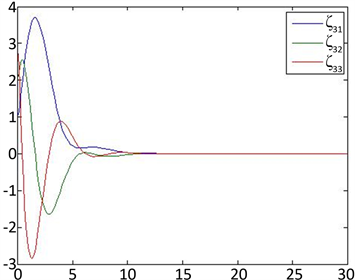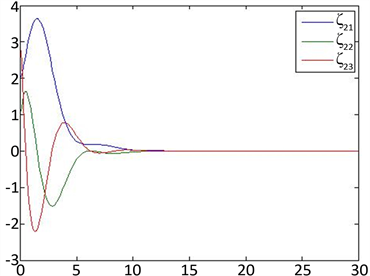(a) 特征值 ${\lambda }_{2}=1$ ，初值为 $\left({\zeta }_{21},{\zeta }_{22},{\zeta }_{23}\right)=\left(2,1,3\right)$ 时系统(8)的解的图像 (b) 特征值 ${\lambda }_{3}=2$ ，初值为 $\left({\zeta }_{31},{\zeta }_{32},{\zeta }_{33}\right)=\left(1,2,3\right)$ 时系统(8)的解的图像

Figure 1. The trajectories of system (8) when $\tau =\frac{1}{4}$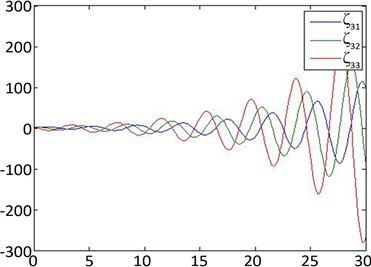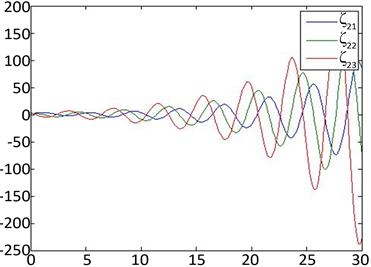(c) 特征值 ${\lambda }_{2}=1$ ，初值为 $\left({\zeta }_{21},{\zeta }_{22},{\zeta }_{23}\right)=\left(2,1,3\right)$ 时系统(8)的解的图像 (d) 特征值 ${\lambda }_{3}=2$ ，初值为 $\left({\zeta }_{31},{\zeta }_{32},{\zeta }_{33}\right)=\left(1,2,3\right)$ 时系统(8)的解的图像

Figure 2. The trajectories of system (8) when $\tau =\frac{\text{2}}{\text{3}}$

Higher-Order Consensus in Multi-Agent System with Delay[J]. 应用数学进展, 2017, 06(09): 1098-1104. http://dx.doi.org/10.12677/AAM.2017.69133

1. 1. 陈杰, 方浩, 辛斌. 多智能体系统的协同群集运动控制[M]. 北京: 科学出版社, 2017.

2. 2. 虞文武, 温光辉, 陈冠荣, 曹进德. 多智能体系统分布式协同控制[M]. 北京: 高等教育出版社, 2016: 218-219.

3. 3. Hong, Y., Chen, G. and Bushnell, L. (2008) Technical Communique: Distributed Observers Design for Leader-Following Control of Mul-ti-Agent Networks. Automatica, 44, 846-850.
https://doi.org/10.1016/j.automatica.2007.07.004

4. 4. Hu, J., Hong, Y. and Gao, L. (2007) Tracking Control for Multi-Agent Consensus with an Active Leader and Variable Topology. Automatica, 42, 1177-1182.

5. 5. Ren, W. (2007) Second-Order Consensus Algorithm with Extensions to Switching Topologies and Reference Models. Proceedings of the 2007 American Control Conference, New York, 11-13 July 2007, 1431-1436.

6. 6. Ren, W. (2008) On Consensus Algorithms for Double-Integrator Dynamics. IEEE Transactions on Automatic Control, 53, 1503-1509.
https://doi.org/10.1109/TAC.2008.924961

7. 7. Ren, W. and Atkins, E. (2013) Second-Order Consensus Protocols in Multiple Vehicle Systems with Local Interactions. AIAA Guidance, Navigation, and Control Conference and Exhibit, San Francisco, 15-18 August 2005, 1-13.

8. 8. Ren, W., Moore, K.L. and Chen, Y. (2007) High-Order and Model Reference Consensus Algorithms in Cooperative Control of MultiVehicle Systems. Journal of Dynamic Systems Measurement & Control, 129, 678-688.
https://doi.org/10.1115/1.2764508

9. 9. Yu, W., Chen, G., Ren, W., et al. (2011) Distributed Higher Order Con-sensus Protocols in Multiagent Dynamical Systems. IEEE Transactions on Circuits & Systems I: Regular Papers, 58, 1924-1932.
https://doi.org/10.1109/TCSI.2011.2106032

10. 10. Yu, W., Chen, G., Cao, M., et al. (2013) Delay-Induced Con-sensus and Quasi-Consensus in Multi-Agent Dynamical Systems. IEEE Transactions on Circuits & Systems I: Regular Papers, 60, 2679-2687.
https://doi.org/10.1109/TCSI.2013.2244357

11. 11. Atay, F.M. (2013) The Consensus Problem in Networks with Transmission Delays. Philosophical Transactions of the Royal Society A, 371, 20120460.

12. 12. Gopalsamy, K. (1992) Stability and Oscillations in Delay Differential Equations of Population Dynamics. Springer Netherlands, 214-218.
https://doi.org/10.1007/978-94-015-7920-9

13. 13. Horn, R.A. and Johnson, C.R. (2013) Matrix Analysis. Cam-bridge University Press, Cambridge.